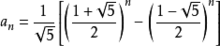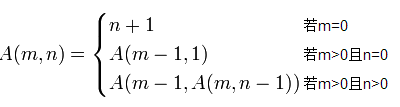Ackerman函数

0
0
01. 云栖社区>
2. 博客>
3. 正文

Ackerman函数

Ackerman函数在许多讲解递归的书中都提到，但似乎又对解题没有太大的意义，暂时不知道了。不过这个东西，是一个数学知识点，暂时收藏于此吧。

Fibonacci数列是一个非常重要，应用非常广的知识点，其递归定义如下：

通项公式Fibonacci数列可以写出通项公式，就是用非递归的方式去下定义。但并非一切的递归函数都能用非递归方式去定义。下面讲一个双递归函数——Ackerman函数，它的复杂性就比Fibonacci数列递归公式要复杂多了。

Ackerman函数有A(n,m)有两个独立的整变量m>=0,n>=0，其定义如下
A(1,0)=2;
A(0,m)=1 m>=0
A(n,0)=n+2 n>=2
A(n,m)=A(A(n-1,m),m-1) n,m>=1
A(n,m)的每一个自变量都定义了一个单变量函数。递归式的第三式定义了函数“加2”。
m=1时,由于A(1,1)=A(A(0,1),0)=A(1,0)=2

A(n,2)=A(A(n-1,2),1)=2A(n-1,2)，因此A(n,2)=2^n

A(n,4)的增长速度已经变得难以想象的快，以至于不能写出一个通项公式来表示这一函数。

A(4)=222…2其中2的层数为65535，这个数有log(A(4))位。所以，对于通常所见到的正整数n，有B(n)<=4

Ackerman函数有A(n,m)有两个独立的整变量m>=0,n>=0，其定义如下
A(1,0)=2;
A(0,m)=1 m>=0
A(n,0)=n+2 n>=2
A(n,m)=A(A(n-1,m),m-1) n,m>=1
A(n,m)的自身变量m的每一个值都定义了一个单变量函数int ack(int m,int n)
{
if(m == 0)
return n+1;
else if(n == 0)
return ack(m-1,1);
else
return ack(m-1,ack(m,n-1));
}

+ 关注

corcosa 14204人浏览